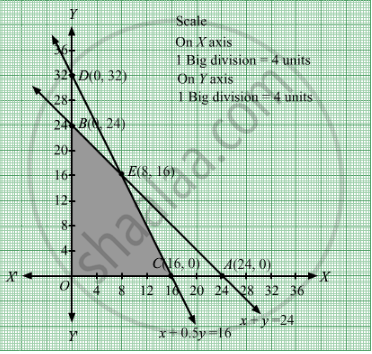# A Small Firm Manufactures Gold Rings and Chains. the Total Number of Rings and Chains Manufactured per Day is at Most 24. - Mathematics

Sum

A small firm manufactures gold rings and chains. The total number of rings and chains manufactured per day is at most 24. It takes 1 hour to make a ring and 30 minutes to make a chain. The maximum number of hours available per day is 16. If the profit on a ring is Rs 300 and that on a chain is Rs 190, find the number of rings and chains that should be manufactured per day, so as to earn the maximum profit. Make it as an LPP and solve it graphically.

#### Solution

Let the firm manufacture gold rings and chains per day.
Number of gold rings and chains cannot be negative.

Therefore, x ≥0 and y ≥0.

It is given that the total number of gold rings and chains manufactured per day is at most 24.

∴ x + y  ≤ 24

The gold ring takes 1 hour to make and chain takes 30 min, that is, 0.5 hour to make.

The maximum number of hours available per day is 16 hours.

∴ + 0.5y ≤ 16

The profit on a ring is Rs 300 and on a chain is Rs 190. Therefore, profit made from gold rings and chains is Rs 300x and Rs 190y respectively.

∴ Total profit, Z = 300x + 190y

Thus, the mathematical formulation of the given problem is:

Maximise Z = 300x + 190y

subject to the constraints

x + y ≤ 24

+ 0.5y ≤ 16

xy ≥0

First we will convert inequations into equations as follows:
x + y = 24, x + 0.5y = 16, x = 0 and y = 0

Region represented by x + y ≤ 24:
The line x + y = 24 meets the coordinate axes at A(24, 0) and $B\left( 0, 24 \right)$  respectively. By joining these points we obtain the line x + y = 24. Clearly (0,0) satisfies the x + y = 24. So, the region which contains the origin represents the solution set of the inequation
x + y ≤ 24.

Region represented by + 0.5y ≤ 16:
The line x + 0.5y = 16 meets the coordinate axes at C(16, 0) and  $D\left( 0, 32 \right)$

respectively. By joining these points we obtain the line x + 0.5y = 16. Clearly (0,0) satisfies the inequation + 0.5y ≤ 16. So,the region which contains the origin represents the solution set of the inequation + 0.5y ≤ 16.

Region represented by ≥ 0 and y ≥ 0:
Since, every point in the first quadrant satisfies these inequations. So, the first quadrant is the region represented by the inequations x ≥ 0, and y ≥ 0.
The feasible region determined by the system of constraints  x + y ≤ 24, + 0.5y ≤ 16, x ≥ 0 and y ≥ 0 are as follows.

The feasible region determined by the system
constraints is as follows:The corner points are O(0, 0), C(16, 0), E(8, 16) and B(0, 24).

The value of Z at these corner points are as follows:

 Corner point Z = 300x + 190y O(0, 0) 0 C(16, 0) 4800 E(8, 16) 5440 B(0, 24) 4560

Thus, the maximum value of Z is 5440 at E(8, 16).

Thus, 8 gold rings and 16 chains should be manufactured per day to maximise the profits.

Concept: Graphical Method of Solving Linear Programming Problems
Is there an error in this question or solution?

#### APPEARS IN

RD Sharma Class 12 Maths
Chapter 30 Linear programming
Exercise 30.4 | Q 38 | Page 55